Class 8 RD Sharma Solutions – Chapter 3 Squares and Square Roots – Exercise 3.3 | Set 1

• Difficulty Level : Easy
• Last Updated : 06 Apr, 2021

(i) 25

Solution:

Here, we will break 25 in as between one’s and ten’s position as a = 2 and b = 5. Now,

Step 1: Make 3 columns and write the values of a², 2ab, b² in these columns.

Step 2: Underline the unit digit of b² and add its ten’s digit(if any) to 2ab.

Step 3: Now Underline the unit digit of column 2 and add its ten’s digit(if any) to a²

Step 4: Now underline the number in column 1.

Step 5: Now write the underlined digits respectively as the square.

252 =  625

Now using multiplication,

252 = 25 x 25 = 625

Because we have the same result in both the methods hence our result is verified.

(ii) 37

Solution:

Here, a = 3, b = 7

Now write the underlined digits respectively as the square.

372 = 1369

Now using multiplication,

372 = 37 x 37 = 1369

Because we have the same result in both the methods hence our result is verified.

(iii) 54

Solution:

Here, a = 5, b = 4.

Now write the underlined digits respectively as the square.

542 = 2916

Now using multiplication,

542 = 54 x 54 = 2916

Because we have the same result in both the methods hence our result is verified.

(iv) 71

Solution:

Here, a = 7, b = 1.

Now write the underlined digits respectively as the square.

712 = 5041

Now using multiplication,

712 = 71 x 71 = 5041

Because we have the same result in both the methods hence our result is verified.

(v) 96

Solution:

Here, a = 9, b = 6.

Now write the underlined digits respectively as the square.

962 = 9216

Now using multiplication,

962 = 96 x 96 = 9216.

Because we have the same result in both the methods hence our result is verified.

(i) 98

Solution:

Because, 98² = 9604

Draw a square table with equal no. of rows and columns as the no. of digits are.

Now divide each block in two parts.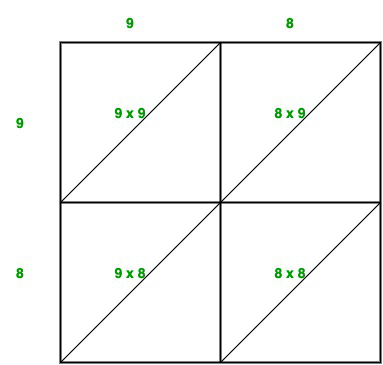Now, write the digits as depicted, and we have to store the values as provided in each block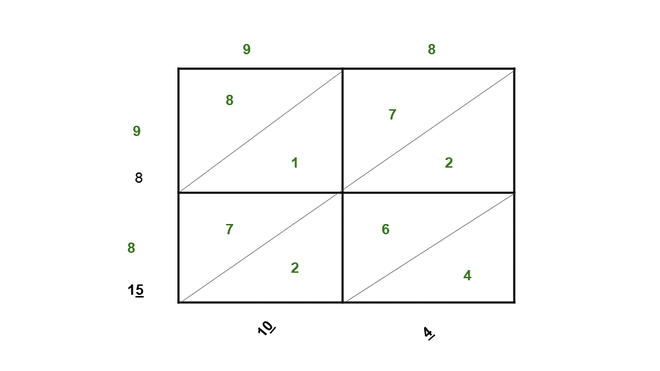Now Store the values as shown and add them as per the subdivision we have made, i.e., 4, 2 + 6 + 2, 7 + 1 + 7, 8 and the previous carry(i.e., take only one unit’s digit and transfer the other as carry).

Now, write the underlined(unit’s digit), as the square of the number

982 = 9604

(ii) 273

Solution:

Because, 2732 = 74529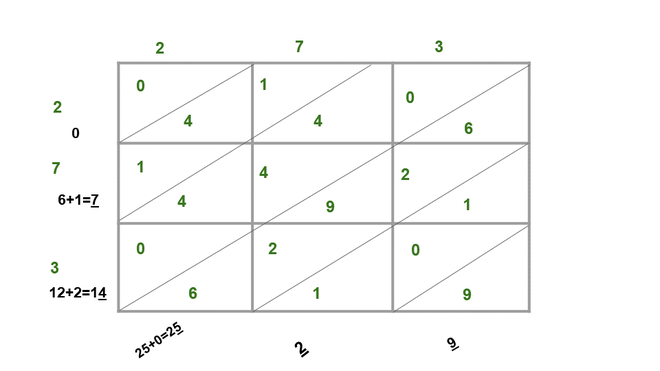Now, write the underlined(unit’s digit), as the square of the number

2732 = 74529

(iii) 348

Solution:

Because, 3482 = 121104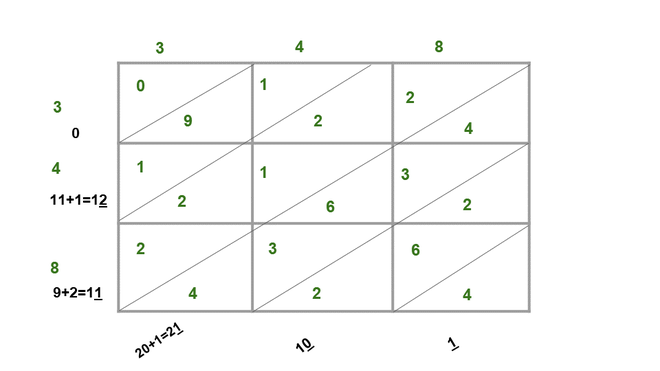Now, write the unit’s digit as the square of 348,

3482 = 121104

(iv) 295

Solution:

Because, 2952 = 87025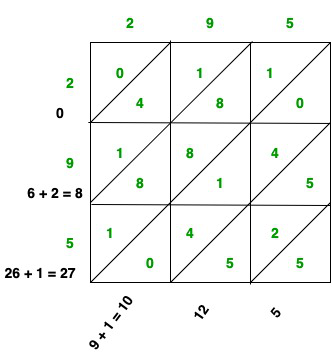Now, write each one’s digit as the square of 295,

2952 = 87025

(v) 171

Solution:

Because, 1712 = 29241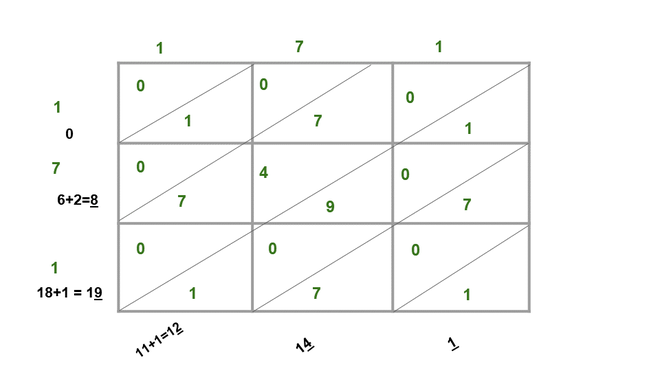Now, write each one’s digit as the square of 171,

1712 = 29241

(i) 127

Solution:

Here let’s take a = 120, b = 7

1272 = (120 + 7)2 = 1202 + (2 x 120 x 7) + 72

= (120 x 120) + 1680 + (7 x 7)

= 14400 + 1680 + 49

= 16129

Alternatively:

We could also take a = 100, b = 27,

1272 = 10000 + 5400 + 729  = 16129

Thus, 1272 = 16129

(ii) 503

Solution:

Here, let’s take a = 500, b = 3.

5032 = (500 + 3)2 = 5002 + (2 x 500 x 3) + 32

= 250000 + 3000 + 9

= 253009

Alternatively:

5032 = 503 x 503 = 253009

Thus, 5032 = 253009

(iii) 451

Solution:

Here, let’s take a = 400, b = 51.

4512 = (400 + 51)2 = 4002 + (2 x 400 x 51) + 512

= 160000 + 40800 + 2601

= 203401

Alternatively:

451² = 451 x 451 = 203401

451² = 203401

(iv) 862

Solution:

Here, let’s take a = 800, b = 62.

8622 = (800 + 62)2 = 8002 + (2 x 800 x 62) + 622

= 640000 + 99200 + 3844

= 743044

Alternatively:

862² = 862 x 862 = 743044

862² = 743044

(v) 265

Solution:

Here, let’s take a = 200, b = 65.

2652 = (200 + 65)2 = 2002 + (2 x 200 x 65) + 652

= 40000 + 26000 + 4225

= 70225

Alternatively:

265² = 265 x 265 = 70225

265² = 70225

Chapter 3 Squares and Square Roots – Exercise 3.3 | Set 2

My Personal Notes arrow_drop_up